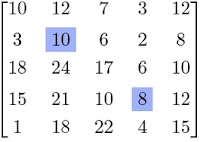## Posts

Showing posts from December, 2011

### Number of digits in 125^100

Source: Asked to me by Suman Kalyan Bera (M.Tech  Student IIT Delhi) on contact page Problem: How many digits does the number 125^100 have? Very interesting problem and equally interesting solution :) Update (25 December 2011): You are not allowed to use your high school notes on values of log_10(2) or log_10(5) Solution: Posted by me in comments!

### Array Problems - Contiguous Sum and Distinct Elements

Source: Posted by Algo Muse on contact page . Also posted on Algo Muse (December 2011) http://www.algomuse.appspot.com Problem: Two definitions: 1) Contiguous t-sum problem Given an array A[1..n] and a number t as input, we want to find out if there exists a sub-array whose sum is t. For example, if the following array and t=8 is the input, the answer is YES since it contains the sub-array A[2..4] whose sum is t.  1 4 -1 5 -8 5 -6 3 10 3  2) Distinct-elements problem Given an array A[1..n], find out if all the elements in the array are distinct. Return YES if all the numbers are distinct NO otherwise. Real Problem: Suppose we are given an algorithm that solves the t-sum problem in O(n) time. Design an algorithm that solves the distinct-elements problem in O(n) time. Update (2nd January 2011): Solution: Posted by Yashoteja (CSE IITB Alumnus, Microsoft Research RA) and Pseudonymous in comment. ThanksSource: Quantnet Interview Questions Problem: If a car passes at the crosswalk on average every 10 seconds and you need 20 seconds to pass the road, how long does it take you on average to cross the road? Note that since the problem is not very well specified, make reasonable assumptions to solve it. It would be fun if before solving mathematically, you try and guess a time estimate. :-) Update (3rd January 2011): Solution posted by Santosh Ananthakrishnan (EE Final Year DD Student IIT Bombay) and Animesh Saxena (Manager at Karvy Private Wealth) in comments!

### Shortest Curve dividing Equilateral Triangle

Source: Asked to me by Dinesh Krithivasan (IITM Alumnus, Phd University of Michigan, Senior Qualcomm Engineer) Finally a geometry problem for the blog. :) Thanks Dinesh! :) Problem : We have an equilateral triangle ABC of unit side length. We want to find a curve C of the smallest length that cuts this triangle into 2 halves of equal area. Obviously, the altitude of length sqrt(3)/2 will do the job but can we do better? Note that there is no other restriction on C - it need not pass through any of the triangle vertices for instance.